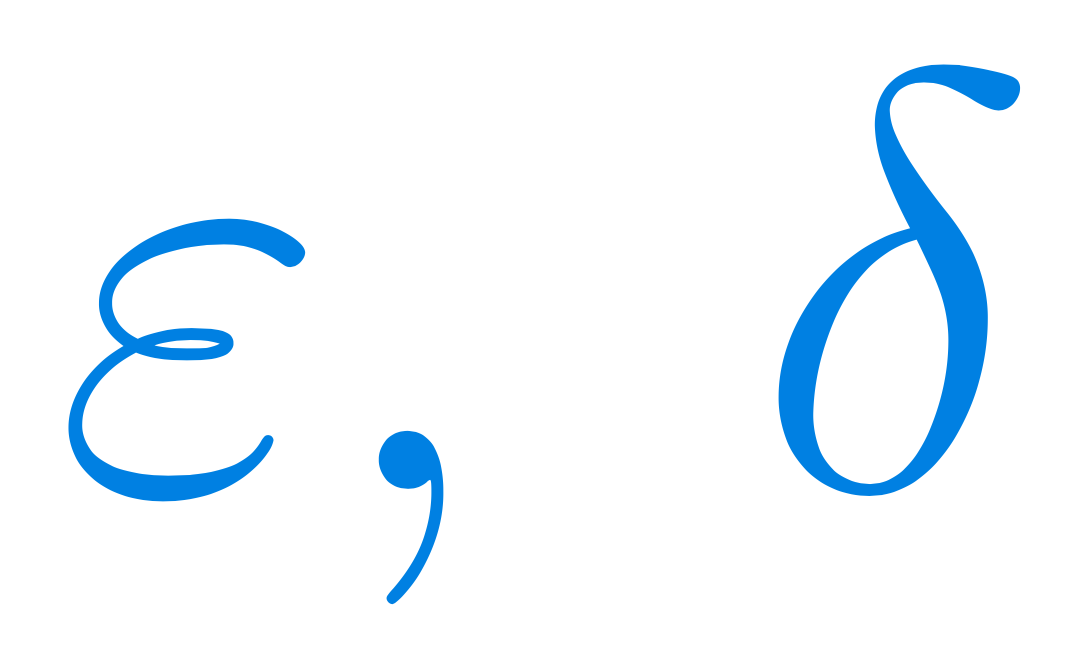Select Page

# Calculus 1, part 1 of 2: Limits and continuity

#### A total of 60 hours of lectures

This is an academic level course for university and college engineering. Due to its size, it is divided into two parts. This page describes the first of those two parts.#### Level - Intermediate

You need to be familiar with the contents of the four Precalculus courses to comfortably follow along in this course.

#### Curriculum

Make sure that you check with your professor what parts of the course you will need for your exam. Such things vary from country to country, from university to university, and they can even vary from year to year at the same university.

# Calculus 1, part 1 of 2

h

## Get the outline

A detailed list of all the lectures in part 1 of the course, including which theorems will be discussed and which problems will be solved. If you are looking for a particular kind of problem or a particular concept, this is where you should look first.## Get Calculus 1 part 1 on Udemy

When you buy the course on Udemy, you get access to it for life. There is just a one-time fee. The prices do vary a lot on Udemy, but if you use our link by clicking on this panel, you will get the best current price. See also our page on “coupon codes” in the menu (the current code is TPOT_DEC23).

## Course Objectives & Outcomes for part 1

Z
How to solve problems concerning limits and continuity of real-valued functions of 1 variable (illustrated with 491 solved problems) and why these methods work.
Z
Arithmetic on the extended reals, and various types of indeterminate forms.
Z
Number sequences and their convergence or divergence; the epsilon-definition of limits of sequences, with illustrations and examples; accumulation points.
Z
Squeeze Theorem for sequences
Z
The concept of a finite limit of a real-valued function of one real variable in a point: Cauchy’s definition, Heine’s definition; proof of their equivalence.
Z
Limit of the sum, difference, product, quotient of two functions; limit of composition of two functions.
Z
Limits and continuity of elementary functions (polynomials, rational f., trigonometric and inverse trigonometric f., exponential, logarithmic and power f.).
Z
Some standard limits in the infinity: a comparison of polynomial growth (more generally: growth described by power f.), exponential, and logarithmic growth.
Z
Starting thinking about plotting functions: domain, range, behaviour around accumulation points outside the domain, asymptotes (vertical, horizontal, slant).
Z
The structure and properties of the set of real numbers as an ordered field with the Axiom of Completeness, and consequences of this definition.
Z
Supremum, infimum, and a reformulation of the Axiom of Completeness in these terms.
Z
Getting new limits from old limits: limit of the sum, difference, product, quotient, etc, of two sequences, with illustrations, formal proofs, and examples.
Z
Squeeze Theorem for functions
Z
Limits at infinity and infinite limits of functions: Cauchy’s definition (epsilon-delta) and Heine’s definition (sequential) of such limits; their equivalence.
Z
Properties of continuous functions: The Boundedness Theorem, The Max-Min Theorem, The Intermediate-Value Theorem.
Z
Some standard limits in zero: sin(x)/x, tan(x)/x, (e^x-1)/x, ln(x+1)/x and a glimpse into their future applications in Differential Calculus.
Z
Continuous extensions and removable discontinuities; examples of discontinuous functions in one, several, or even infinitely many points in the domain.
Z
An introduction to more advanced topics: Cauchy sequences and their convergence; a word about complete spaces; limits and continuity in metric spaces.